# Adding time and date calculated metrics

Follow

Time and date are two of the most common choices for comparison. These two calculations aim to make comparing time with reference numbers as easy as possible. Both of these calculated metrics are found in the Calculation engine menu (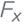).

There are two calculated metrics used for comparing results by time and date:

## Time comparison calculated metric

A time comparison calculated metric is used when you need to compare your results against a dynamic reference number. For example:
• Results against the previous month.
• Results against the same month in the previous year.
• Results against a rolling 12-month average.

A time comparison calculated metric can only reference metric from the original dataset, not any calculated metric. But you can still use them in calculated metrics, such as growth rates.

To use a calculated metric, you must enter the following information:
• Name of your time-comparison calculated metric.
• The metric.
• Your date field. The date field is a date attribute for a specific event. In the example below, the calculation is measuring profit by when the product was shipped.
• The offset level. This is the time category that will appear on your chart and what your results are measured against.
• The offset start and end. The offset start and end points are the values the calculations begins and stops measuring at. For example, an offset start point of one would begin measuring at the first Year value. An offset end point of three would stop measuring at the third Year value.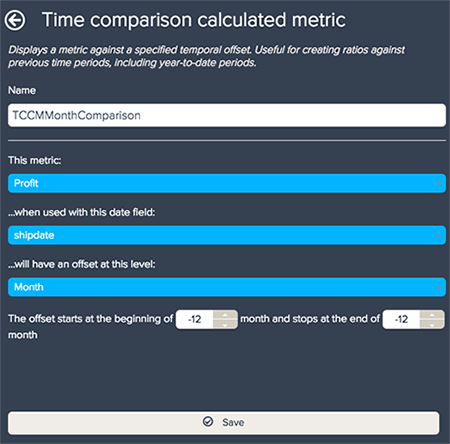On the line chart below, you can see the time comparison calculated metric and the profit. Because the offset start point is twelve, the first twelve months of the time comparison metric are null.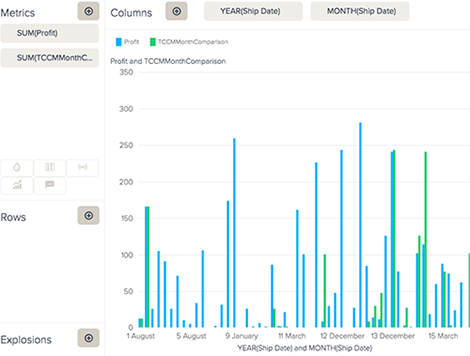Time comparison calculated metrics are particularly useful in metric comparison visuals such as KPI, gauge, and bullet charts (see Comparing metrics). Time comparison calculated metrics are dynamic, so they will react to interactions and filters. For example with date filtering, the offset will remain the same no matter what time period the viewer looks at.

## Date range calculated metrics

Date range calculated metrics create totals for a specified period of time. They are particularly useful for looking at metrics for ranges that don't fit neatly into a calendar period, such as 'the last seven days', 'the previous seven days', or dates that can move around year-to-year like Easter or the summer sales.

Note: Date range calculated metrics are different than attribute date ranges. For information on date ranges used on attributes, see Editing date ranges.

When specifying the period for your date range calculated metric, you have as many options as with using the date range option on queries. There are two different date range calculated metric options: simple and advanced.

In the simple option, you can select from a list of named periods or the Custom option to select a specific calendar start and end day, month, or year.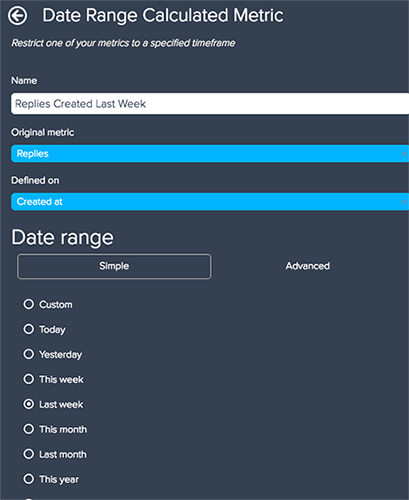For the advanced option, you can select separate beginning and end dates. For each beginning and end date, you can select from a list of named periods, enter in a calendar date, view all history, or select a rolling period. In a rolling period, you can select options like seven days in the past or a year in the future.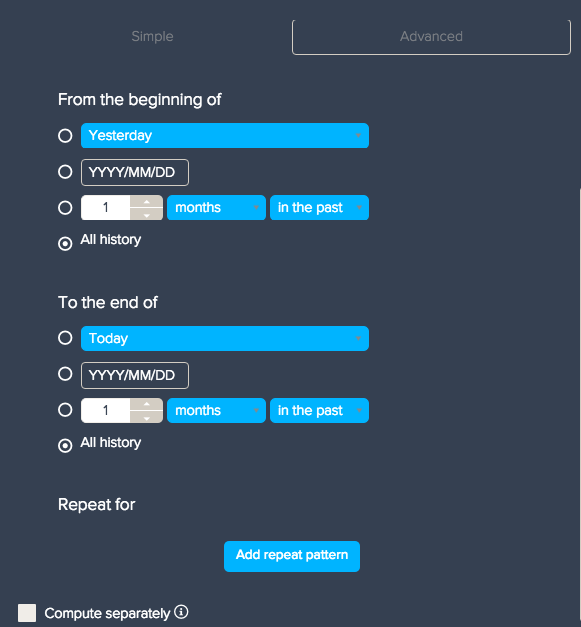You can also repeat the selected range and compute the date range calculated metric separately from your other calculated metrics. This option is useful if you are using metrics or attributes that are in other calculated metrics and attributes. If you select Compute separately, there will be a separate SQL calculation for the query, so it will not conflict with any other calculated metrics.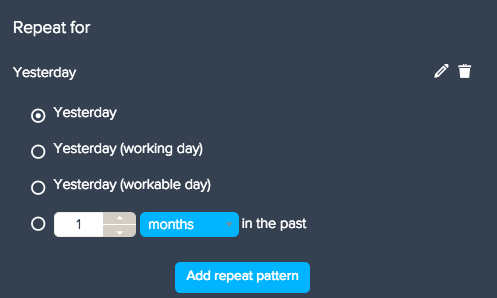Was this article helpful?
1 out of 1 found this helpful
Have more questions? Submit a request

#### 0 Comments

Please sign in to leave a comment.